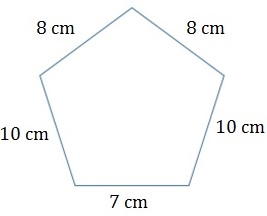# Perimeter of a Polygon

A polygon is a 2-dimensional figure formed by straight sides. The polygon with least number of sides is the triangle. Squares, rectangles, parallelograms, trapezoids are four sided polygons. A polygon with five sides is called a pentagon and a polygon of eight sides is called an octagon and so on.

A polygon with all sides equal and all angles equal is a regular polygon, otherwise it is an irregular polygon.

The perimeter of the polygon is the sum total of the lengths of its sides.

Formula

If the length of side of a regular polygon of n sides is l, then its perimeter is given by nl

Find the perimeter of the given polygon.### Solution

Step 1:

The perimeter of any shape is the sum of the lengths of its sides.

Step 2:

So perimeter of given polygon =

8 cm + 8 cm + 10 cm + 10 cm + 7 cm = 43 cm

If the length of side of a regular polygon of 8 sides is 6 m, then find its perimeter.

### Solution

Step 1:

The length of side of a regular polygon of 8 sides = 6 m,

Step 2:

Perimeter of regular polygon = nl = no. of sides × length of its side

= 8 × 6 m = 48 m